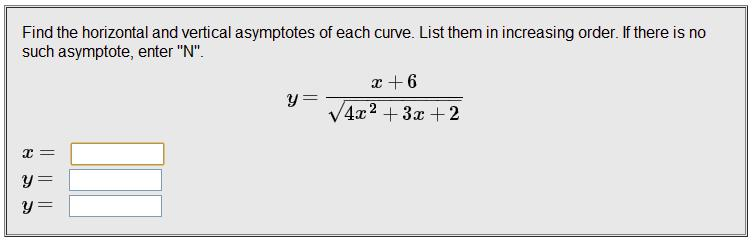# Finding Horizontal & Vertical Asymptotes Of A Curve

## Homework StatementNone

## The Attempt at a Solution

I was able to find a vertical asymptote at x=-3/8 by setting the denominator to 0 and using the quadratic formula to find the roots. However, I am unsure of how to find the horizontal asymptotes, and I am not 100% confident that my vertical asymptote is correct. The confusion that I have here is with the square root in the denominator.

Staff Emeritus
Homework Helper
Gold Member

## Homework StatementNone

## The Attempt at a Solution

I was able to find a vertical asymptote at x=-3/8 by setting the denominator to 0 and using the quadratic formula to find the roots. However, I am unsure of how to find the horizontal asymptotes, and I am not 100% confident that my vertical asymptote is correct. The confusion that I have here is with the square root in the denominator.
Recheck that result you get from "setting the denominator to 0 and using the quadratic formula to find the roots".

For the horizontal asymptote(s), find the limit of your function as x → ± ∞ .